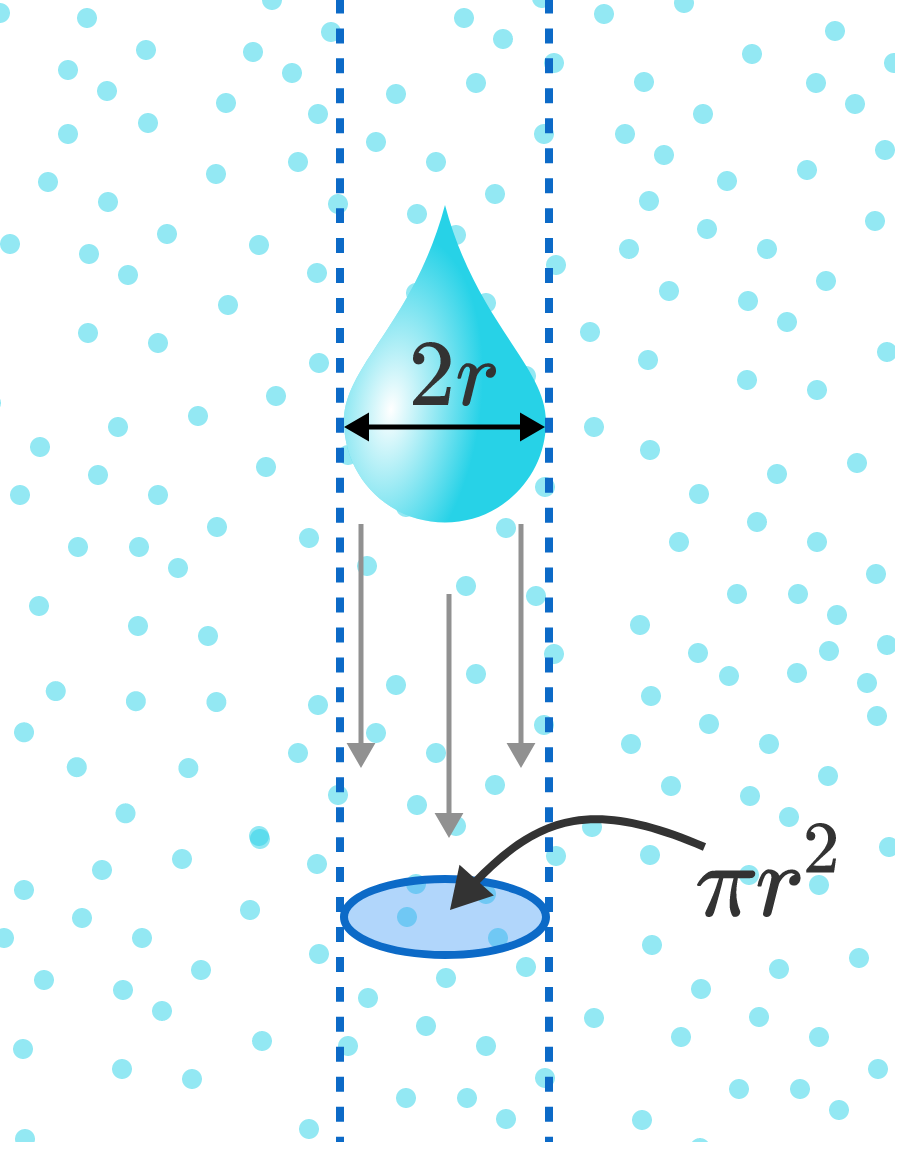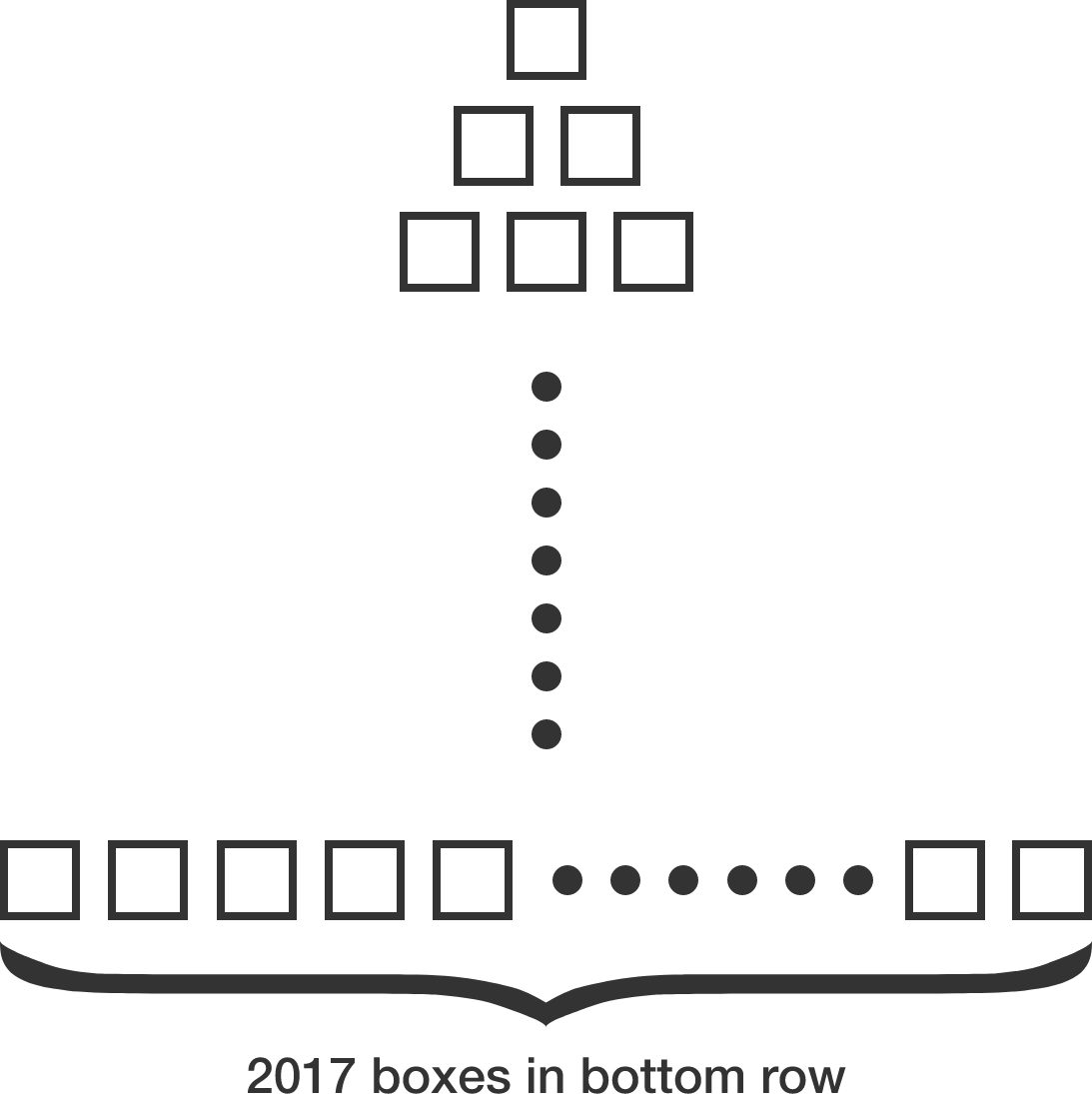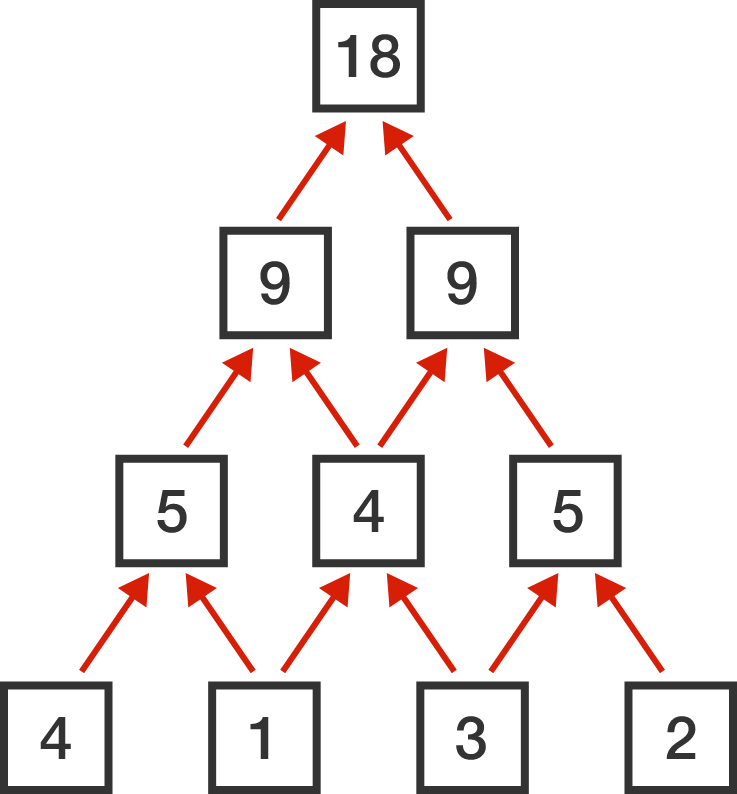# Problems of the Week

Contribute a problem

Write one number in each red circle so that the sum of the numbers along each blue, diagonal line is always the same number $x.$

Find the maximum value of $x$.Consider the following model:

A raindrop falls from rest with a small initial mass, $m_0.$ As it falls, it accumulates mass by absorbing the smaller water drops in its path, which we approximate by the uniform density $\rho_\textrm{atm}.$

Throughout the fall, it maintains the same characteristic shape defined by its width, $2r,$ which increases as it picks up mass at the rate $\dot{m} = \rho_\textrm{atm}\pi r^2 v$ (in time $dt$, it sweeps out a vertical cylinder of height $vdt$).

How will its velocity depend on time? Surprisingly, this complicated problem has a simple solution: after a short time, the raindrop will accelerate at the constant rate $a=\frac gK$ (convince yourself of this!).

What is the value of $K?$


Details and Assumptions:

• Neglect the effects of air resistance, wind speed, the density of air, or any other atmospheric factor except those stated in the problem.

Inspired by a similar problem by Tapas Mazumdar

How many real $x$ satisfying $0 \leq x \leq 2017$ are there such that $x \sin(\pi x)$ is an integer?

Bonus: Can you come up with a simple formula for how many $0 \leq x \leq n$ there are such that $x \sin(\pi x)$ is an integer, where $n$ is a positive integer?

Consider a pyramid with 2017 rows made up of empty boxes. The first (top) row has 1 box, and each successive row has an additional box so that the $2017^\text{th},$ bottom row has 2017 boxes.First, place each of the first 2017 positive integers into the boxes in the bottom row. Then, each empty box in the $2016^\text{th}$ row is filled with the sum of the two numbers beneath it. Then, each successively higher row of boxes is filled in the same way.

Let $M$ and $m$ be the maximum and minimum possible values, respectively, of the single box on the top. Then $M + m = a \times 2^b,$ where $a$ and $b$ are positive integers such that $b$ is as large as possible. Find the value of $a + b.$

Note: Below is an example of how a pyramid of 4 rows could be filled.Let $\sigma$ be a permutation of $\{1, 2, 3, \ldots, 100\}$. Then there exists a smallest positive integer $f(\sigma)$ such that $\sigma^{f(\sigma)} = \text{id}$, where $\text{id}$ is the identity permutation.

What is the largest value of $f(\sigma)$ over all $\sigma?$


Note: $\sigma^k$ is $\sigma$ applied $k$ times in succession.

×